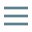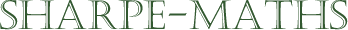Previous topic:
Factorizing expressions
Current topic:
Next topic:
When you first look at the quadratic expression $x^2+3x+2$, you are likely to think that it cannot be factorised; that it is not the result of a multiplication, but it is fairly straightforward to demonstrate that this is not the case.
\begin{aligned} &\big(x+1 \big)\big(x+2 \big)\\ & = x^2+2x+1x+2 \\ &= x^2+3x+2 \end{aligned}
From the calculation above it is clear that $(x+1)$ and $(x+2)$ are both factors of $x^2+3x+2$.